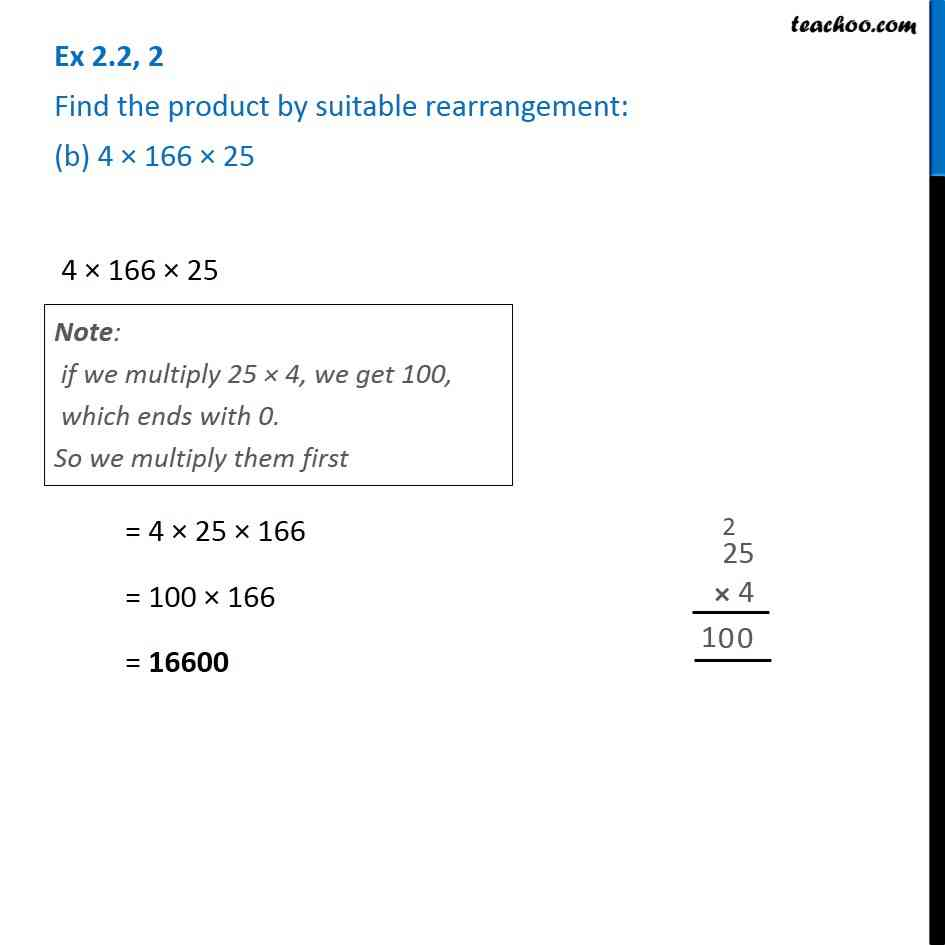Ex 2.2

Chapter 2 Class 6 Whole Numbers
Serial order wiseGet live Maths 1-on-1 Classs - Class 6 to 12

### Transcript

Ex 2.2, 2 Find the product by suitable rearrangement: (b) 4 × 166 × 25 4 × 166 × 25 = 4 × 25 × 166 = 100 × 166 = 16600 Note: if we multiply 25 × 4, we get 100, which ends with 0. So we multiply them first